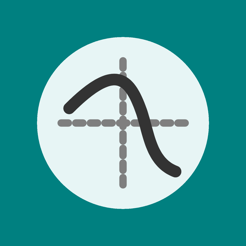## Screenshots

•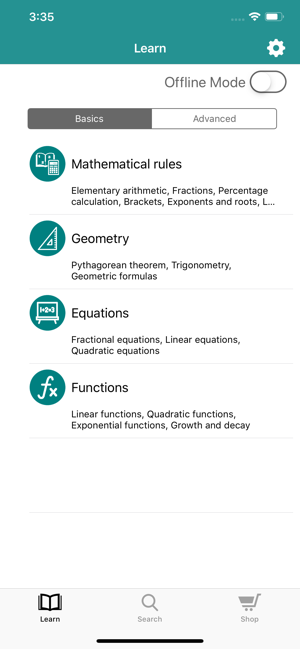•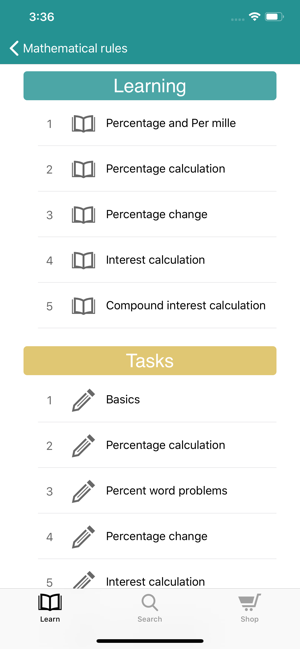•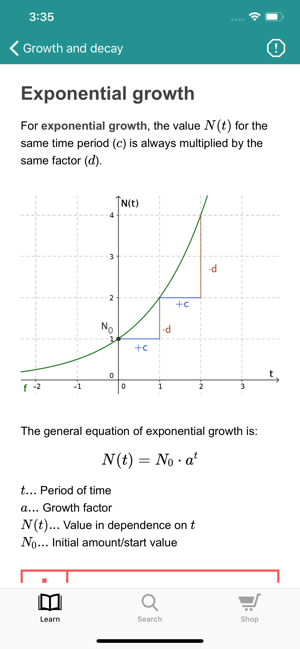•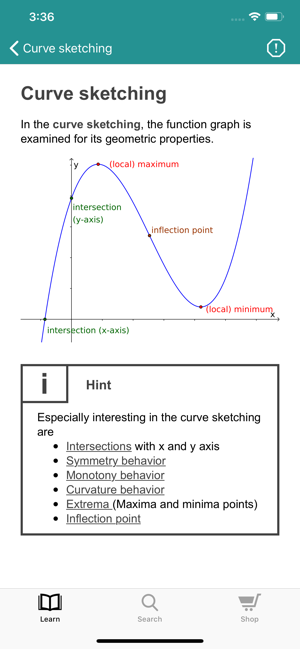•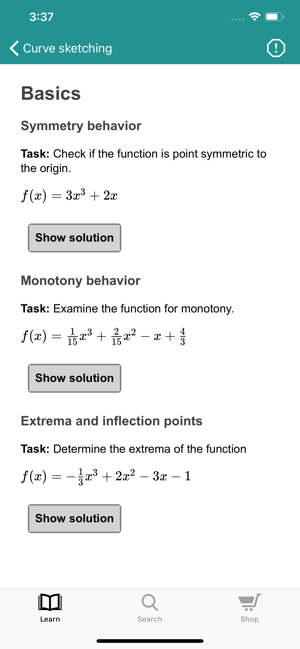## Description

"What is a linear function?", "How to solve a quadratic equation?", "What is the Pythagorean theorem all about?"

Math Wiki provides numerous exercises and articles in the field of mathematics, which are helpful to better understand the foundations of mathematics from 7th grade upwards. The app is also useful in preparation for middle school exams, high school and college math.

Math Wiki includes the following features:

• portable and compact reference work for math
• glossary of mathematical terms
• numerous foundations from 7th grade
• ideal for learning, reference and practice
• simple and detailed explanations with lots of examples for better understanding
• exercises on every topic
• clear structuring with subtopics
• search option
• suitable for high school graduation and middle school exams

Math Wiki has many explanations of important topics of mathematics which are described in detail. In addition to the explanations there are examples and lots of tasks with solutions. Learn, repeat or practice math anytime and anywhere with our wiki and encyclopedia of mathematics.

The following topics are already included:

Arithmetic / laws of arithmetic / mathematical rules
• Elementary arithmetic
• Fractions
• Percentage calculation
• Brackets
• Exponents and roots
• Logarithms

Geometry
• Geometric formulas
• Pythagorean theorem
• Trigonometry

Equations
• Fractional equations
• Linear equations
• Systems of linear equations
• Solve equations

Functions
• Linear functions
• Sine and cosine
• Trigonometric functions
• Exponential functions
• Growth and decay
• Real functions
• Exponential functions
• Rational functions
• Family of curves

Stochastic
• Random experiments and events
• Probability
• Calculating probability
• Tree diagrams
• Combinatorics
• Statistics

Differential calculus
• Differentiability and derivative
• Derivative rules
• Applying the derivative
• Curve sketching
• Applications of differential calculus

Integral calculus
• Indefinite integral
• Integration rules
• Definite integrals
• Calculating areas with integrals
• Applications of integral calculus

Analytical geometry
• Vectors
• Vector algebra
• Lines in three dimensions
• Relative position of lines
• Planes
• Relative position of planes

We strive to constantly expand the app and add new items and tasks.
Feel free to send us your feedback, requests, topics and suggestions to math@lakschool.com.

Version 1.1.2

Bug fixes

## Information

Provider
Lenard Kratky
Size
25.5 MB
Category
Education
Compatibility

Requires iOS 9.0 or later. Compatible with iPhone, iPad and iPod touch.

Languages

English, German, Russian

Age Rating
4+
Price
Free
In-App Purchases
2. MatheWiki Aufgaben Rs 600.00
•